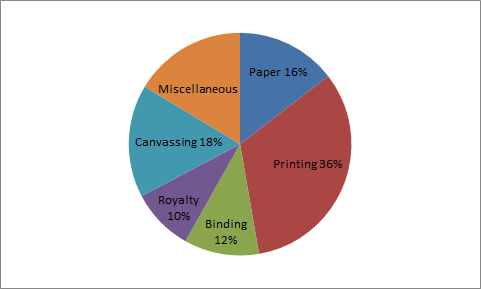# Data Interpretation - Pie Chart

>>>>>>>>Pie Chart

Instructions:

The pie chart given below shows the expenditure incurred in bringing out a book, by a publisher:study the graph and answer the questions 21-24  given below

What is the central angle showing the cost of paper?

• A

16°• B

32°• C

28.8°• D

57.6°• Option : D
• Explanation :

Required Central Angle = (16/100) * 360 = 57.6° Click on Discuss to view users comments.

Instructions:

The pie chart given below shows the expenditure incurred in bringing out a book, by a publisher:study the graph and answer the questions 21-24  given below

• A

Rs. 6500• B

Rs. 2340• C

Rs. 4680• D

Rs. 7840• Option : A
• Explanation : Let the royalty be Rs. x Then.

36:10 : : 23400 : x

So, x = (10 * 23400)/36 = Rs. 6500

Instructions:

The pie chart given below shows the expenditure incurred in bringing out a book, by a publisher:study the graph and answer the questions 21-24  given below

• A

Rs. 8000• B

Rs. 14400• C

Rs. 46800• D

Rs. 40500• Option : D
• Explanation : Let the expenditure on canvassing be Rs. x

Then, 8:18 : : 18000:x

So, x = (18*18000)/8 = Rs.40500

Instructions:

The pie chart given below shows the expenditure incurred in bringing out a book, by a publisher:study the graph and answer the questions 21-24  given below

• A

8%• B

80%• C

44 4/9%• D

None• Option : C
• Explanation : If canvassing charges are Rs. 18, royalty is Rs. 10.

On Rs. 18. it is less by 8

On Rs. 100, it is less by ((8/18) * 100)%

=44 4/9 %

• A• BRelated Quiz.
Pie Chart